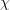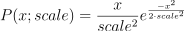/NumPy 1.14

# numpy.random.rayleigh

numpy.random.rayleigh(scale=1.0, size=None)

Draw samples from a Rayleigh distribution.

Theand Weibull distributions are generalizations of the Rayleigh.

Parameters: scale : float or array_like of floats, optional Scale, also equals the mode. Should be >= 0. Default is 1. size : int or tuple of ints, optional Output shape. If the given shape is, e.g., (m, n, k), then m * n * k samples are drawn. If size is None (default), a single value is returned if scale is a scalar. Otherwise, np.array(scale).size samples are drawn. out : ndarray or scalar Drawn samples from the parameterized Rayleigh distribution.

#### Notes

The probability density function for the Rayleigh distribution isThe Rayleigh distribution would arise, for example, if the East and North components of the wind velocity had identical zero-mean Gaussian distributions. Then the wind speed would have a Rayleigh distribution.

#### References

 [R519520] Brighton Webs Ltd., “Rayleigh Distribution,” http://www.brighton-webs.co.uk/distributions/rayleigh.asp
 [R520520] Wikipedia, “Rayleigh distribution” http://en.wikipedia.org/wiki/Rayleigh_distribution

#### Examples

Draw values from the distribution and plot the histogram

>>> values = hist(np.random.rayleigh(3, 100000), bins=200, normed=True)


Wave heights tend to follow a Rayleigh distribution. If the mean wave height is 1 meter, what fraction of waves are likely to be larger than 3 meters?

>>> meanvalue = 1
>>> modevalue = np.sqrt(2 / np.pi) * meanvalue
>>> s = np.random.rayleigh(modevalue, 1000000)


The percentage of waves larger than 3 meters is:

>>> 100.*sum(s>3)/1000000.
0.087300000000000003


© 2008–2017 NumPy Developers
Licensed under the NumPy License.
https://docs.scipy.org/doc/numpy-1.14.2/reference/generated/numpy.random.rayleigh.html